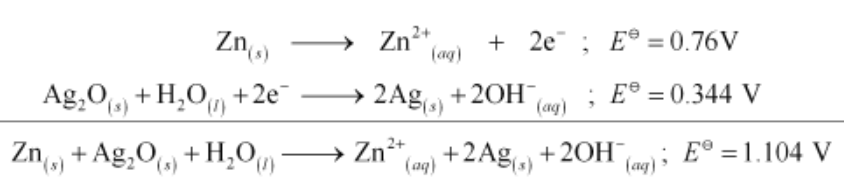# In the button cells widely used in watches and other devices the following reaction takes place:Question:

In the button cells widely used in watches and other devices the following reaction takes place:

Zn(s) + Ag2O(s) + H2O(l) → Zn2+(aq) + 2Ag(s) + 2OH(aq)

Determine $\Delta_{r} G^{\oplus}$ and $E^{\oplus}$ for the reaction.

Solution:$\therefore E^{\ominus}=1.104 \mathrm{~V}$

We know that,

$\Delta_{r} G^{\ominus}=-n \mathrm{~F} E^{\ominus}$

= −2 × 96487 × 1.04

= −213043.296 J

= −213.04 kJ